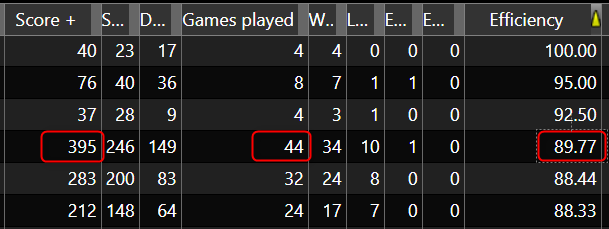Efficiency is a stat to see how efficient a player can be.

Score+ divided by (games played x points max) X 100

395/ (44 X 10) X 100 =

(395 / 440) X 100 =

0.89772727 X 100 =

89.77